Postulates & Operators in Quantum Mechanics

# Postulates & Operators in Quantum Mechanics Notes | Study Physical Chemistry - Chemistry

## Document Description: Postulates & Operators in Quantum Mechanics for Chemistry 2022 is part of Physical Chemistry preparation. The notes and questions for Postulates & Operators in Quantum Mechanics have been prepared according to the Chemistry exam syllabus. Information about Postulates & Operators in Quantum Mechanics covers topics like Postulates of Quantum Mechanics and Postulates & Operators in Quantum Mechanics Example, for Chemistry 2022 Exam. Find important definitions, questions, notes, meanings, examples, exercises and tests below for Postulates & Operators in Quantum Mechanics.

Introduction of Postulates & Operators in Quantum Mechanics in English is available as part of our Physical Chemistry for Chemistry & Postulates & Operators in Quantum Mechanics in Hindi for Physical Chemistry course. Download more important topics related with notes, lectures and mock test series for Chemistry Exam by signing up for free. Chemistry: Postulates & Operators in Quantum Mechanics Notes | Study Physical Chemistry - Chemistry
 1 Crore+ students have signed up on EduRev. Have you?

Postulates of Quantum Mechanics

Here are the Six Postulates of Quantum Mechanics. Postulate 2 contains various Operators use in Quantum Mechanics.

Postulate 1. The state of a quantum mechanical system is completely specified by a function ψ(r, t) that depends on the coordinates of the particle(s) and on time. This function, called the wave function or state function, has the important property that ψ(r, t) ψ(r, t) dτ is the probability that the particle lies in the volume element  located at r at time .
The wavefunction must satisfy certain mathematical conditions because of this probabilistic interpretation. For the case of a single particle, the probability of finding it somewhere is 1, so that we have the normalization condition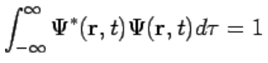(110)
It is customary to also normalize many-particle wavefunctions to 1.2 The wavefunction must also be single-valued, continuous, and finite.

Postulate 2. To every observable in classical mechanics there corresponds a linear, Hermitian operator in quantum mechanics.
This postulate comes about because of the considerations raised in section 3.1.5: if we require that the expectation value of an operator Â is real, then Â must be a Hermitian operator. Some common operators occuring in quantum mechanics are collected in Table 1.
Table 1: Physical observables and their corresponding quantum operators (single particle)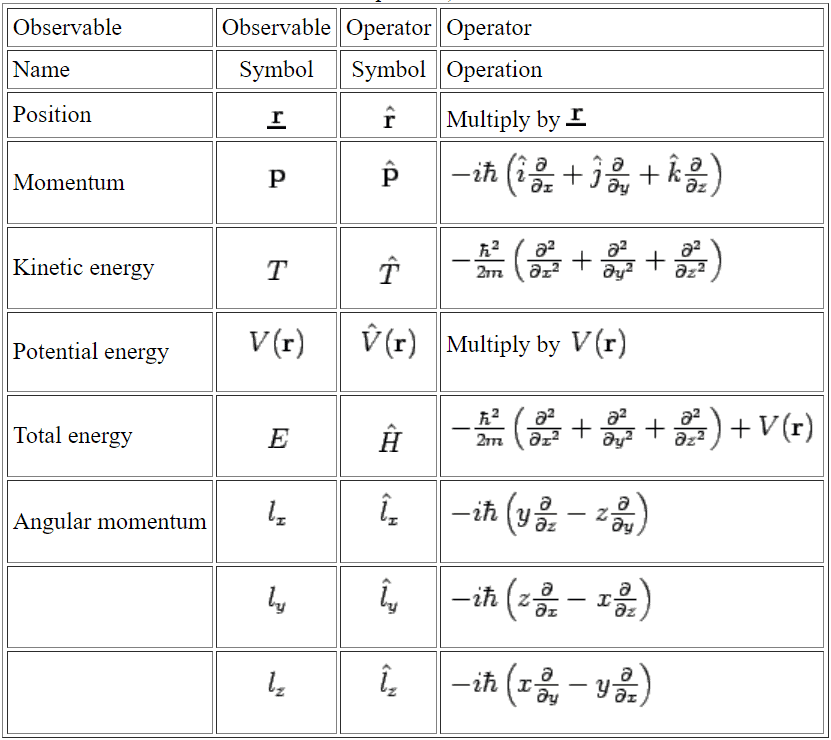Postulate 3. In any measurement of the observable associated with operator Â, the only values that will ever be observed are the eigenvalues a, which satisfy the eigenvalue equation
ÂΨ = aΨ          (111)
This postulate captures the central point of quantum mechanics--the values of dynamical variables can be quantized (although it is still possible to have a continuum of eigenvalues in the case of unbound states). If the system is in an eigenstate of Â with eigenvalue a, then any measurement of the quantity A will yield a.
Although measurements must always yield an eigenvalue, the state does not have to be an eigenstate of Â initially. An arbitrary state can be expanded in the complete set of eigenvectors of Â (ÂΨi = aiΨi) as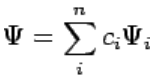(112)
where n may go to infinity. In this case we only know that the measurement of A will yield one of the values ai, but we don't know which one. However, we do know the probability that eigenvalue ai will occur--it is the absolute value squared of the coefficient, |ci|2 (cf. section 3.1.4), leading to the fourth postulate below.
An important second half of the third postulate is that, after measurement of Ψ yields some eigenvalue ai, the wavefunction immediately ``collapses'' into the corresponding eigenstate Ψi (in the case that ai is degenerate, then Ψ becomes the projection of Ψ onto the degenerate subspace). Thus, measurement affects the state of the system. This fact is used in many elaborate experimental tests of quantum mechanics.

Postulate 4. If a system is in a state described by a normalized wave function Ψ, then the average value of the observable corresponding to Â is given by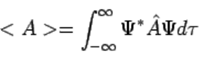(113)

Postulate 5. The wavefunction or state function of a system evolves in time according to the time-dependent Schrödinger equation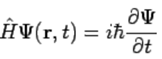(114)
The central equation of quantum mechanics must be accepted as a postulate, as discussed in section 2.2.

Postulate 6. The total wavefunction must be antisymmetric with respect to the interchange of all coordinates of one fermion with those of another. Electronic spin must be included in this set of coordinates.

The document Postulates & Operators in Quantum Mechanics Notes | Study Physical Chemistry - Chemistry is a part of the Chemistry Course Physical Chemistry.
All you need of Chemistry at this link: Chemistry

## Physical Chemistry

67 videos|106 docs|31 tests
 Use Code STAYHOME200 and get INR 200 additional OFF

## Physical Chemistry

67 videos|106 docs|31 tests

Track your progress, build streaks, highlight & save important lessons and more!

,

,

,

,

,

,

,

,

,

,

,

,

,

,

,

,

,

,

,

,

,

;